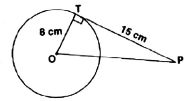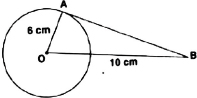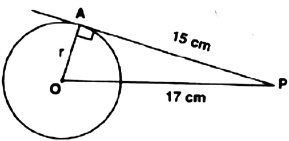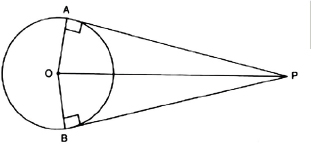# Circles-Sample Papers

Class X Math
Test for Facts that Matter
Total Marks: 25
Total Time: 32 min
1.   In the adjoining figure, PA and PB are tangents from P to a circle with centre. C If ∠APB = 40° then find ∠ACB.
(1 Mark)
2.   In the given figure, PT is a tangent to the circle and O is its centre. Find OP.
(1 Mark)3.   If O is the centre of the circle, then find the length of the tangent AB in the given figure.
(1 Mark)4.   From a point P, the length of the tangent to a circle is 12 cm and distance of P from the centre of the circle is 17 cm, then what is the radius of the circle?
(2 Marks)5.   Prove that the tangents drawn at the ends of a chord of a cricle make equal angles with the chord.
(2 Marks)
6.   Two concentric circle have a common centre O. The chord AB to the bigger circle touches the smaller circle at P. If OP = 3 cm and AB = 8 cm then find the radius of the bigger circle.
(3 Marks)
7.   Given two concentric circle of radii 10 cm and 6 cm. Find the length of the chord of the larger circle which touches the other circle.
(3 Marks)
8.   In a right ΔABC, right angled at B, BC = 5 cm and AB = 12 cm. The cirlce is touching the sides of ΔABC. Find the radius of the circle.
(4 Marks)
9.   Prove that the parallelogram circumscribing a circle is a rhombus.
(3 Marks)
10.   In the following figure, OP is equal to diameter of the circle. Prove that ABP is an equilateral triangle.
(5 Marks)